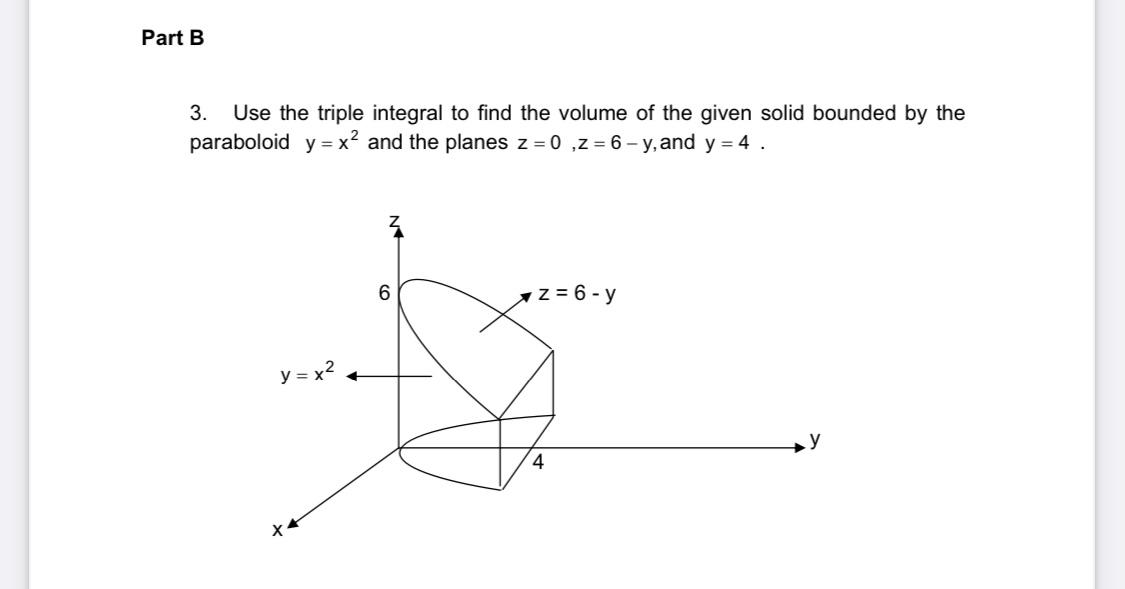### Create an Account

Home / Questions / Part B 3. Use the triple integral to find the volume of the given solid bounded by the par...

# Part B 3. Use the triple integral to find the volume of the given solid bounded by the paraboloid y=x? and the planes z=0,2 = 6 - y, and y = 4 . 6 z = 6 - y y=x2 4 х

Part B 3. Use the triple integral to find the volume of the given solid bounded by the paraboloid y=x? and the planes z=0,2 = 6 - y, and y = 4 . 6 z = 6 - y y=x2 4 хApr 26 2021 View more View LessSubscribe To Get Solution